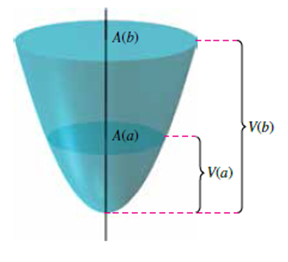# Volume and Surface Area From 1963 to 1986, the volume of the Great Salt Lake approximately tripled while its top surface area approximately doubled. Read the article “Relations between Surface Area and Volume in Lakes” by Daniel Cass and Gerald Wildenberg in The College Mathematics Journal. Then give examples of solids that have “water levels” a and b such that V(b) = 3V(a) and A(b) = 2A(a), where V is volume and A is area (see figure).### Multivariable Calculus

11th Edition
Ron Larson + 1 other
Publisher: Cengage Learning
ISBN: 9781337275378

#### Solutions

Chapter
Section### Multivariable Calculus

11th Edition
Ron Larson + 1 other
Publisher: Cengage Learning
ISBN: 9781337275378
Chapter 14, Problem 13PS
Textbook Problem
1 views

## Volume and Surface Area From 1963 to 1986, the volume of the Great Salt Lake approximately tripled while its top surface area approximately doubled. Read the article “Relations between Surface Area and Volume in Lakes” by Daniel Cass and Gerald Wildenberg in The College Mathematics Journal. Then give examples of solids that have “water levels” a and b such that V(b) = 3V(a) and A(b) = 2A(a), where V is volume and A is area (see figure).This textbook solution is under construction.

### Still sussing out bartleby?

Check out a sample textbook solution.

See a sample solution

#### The Solution to Your Study Problems

Bartleby provides explanations to thousands of textbook problems written by our experts, many with advanced degrees!

Get Started